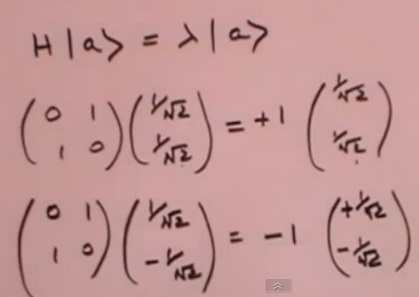Animated Physics - Understanding Photon Polarization

 Energy
 The only thing for sure about energy, is that its a long way away in a very short time. Ie. in one second its 186,000 miles away. A photon is a packet of energy.

 Photons - Polarization
 All photons travel at the speed of light, but when they hit something, its like they were pointed in a particular directon, or spinning around a particular axis. This is called the photon polarization. Polarization can be a direction like up or down, left or right. Polarization can also mean spin in a clockwise or counter-clockwise direction.

 The Planck constant
 The Planck constant is equal to 6.626*10^-34 Joule-Sec. and establishes the relationship between wavelength and energy. A short length = high energy (ultraviolet) and a long length = low energy (heat). To convert from length to energy, take 1240 divided by the nanometer wavelength to get the energy in evolts. For a green (630nm) photon 1240/630nm=1.97ev

Quantum Mechanics Concepts - DrPhysicsA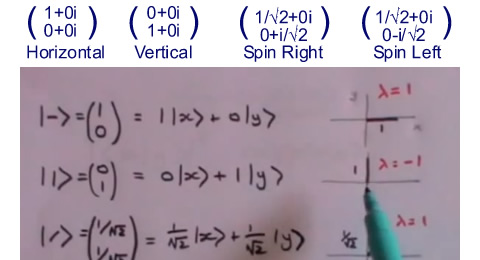This video will provide you with the information to calculate the probability that light polarized in a specific direction will get through a polarizer pointed in a different direction. You will learn to represent a photon of light as an array with two complex numbers in it. The complex conjugate of another array (representing the polarizer angle) can then be multiplied by this array (the photon representation) to give you the probability that the photon will go through the polarizer.     The representation of a photon as a vector, is the polarization component of the Jones Vector. To represent this visually, the real part of the variable is where the polarization is right now. The imaginary part represents where the polarization angle is going. Remember, these angles only represent the amplitude of probability.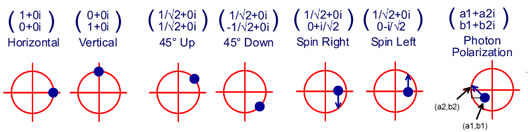0:00 Quantum Mechanics Concepts, Dirac notation and photon polarization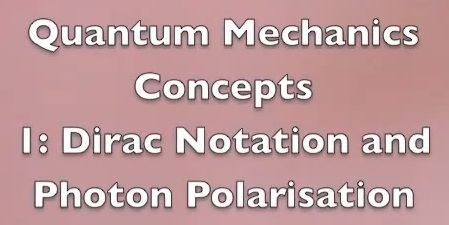1:35 Notations and Mathematics, Ket and Bra vectors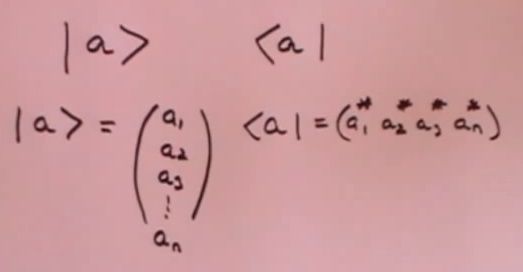3:30 Complex numbers and mapping on a plane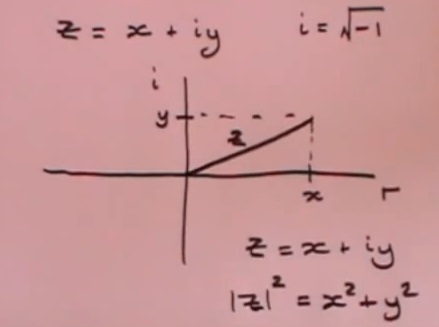5:30 Complex conjugates and some calculated results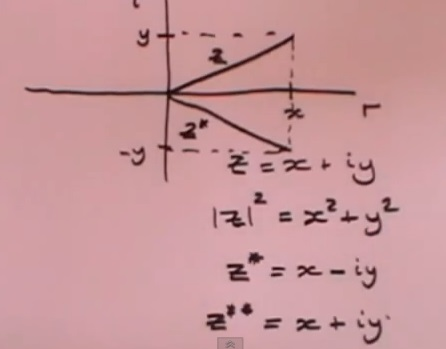9:10 Ket and Bra vector mathematics, addition and scalar multiplication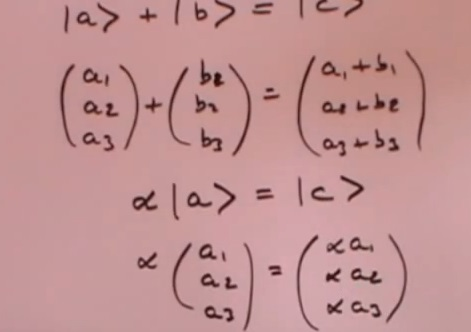11:20 Multiply a Bra vector times a Ket vector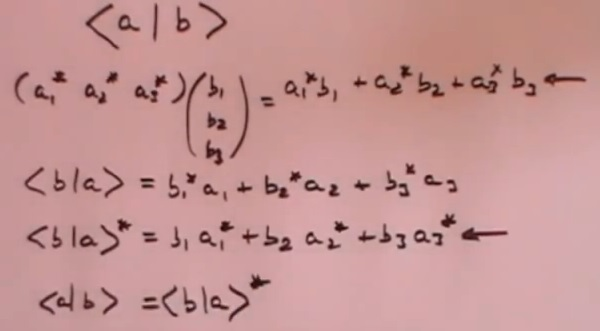14:05 Matrices - Conjugated, Transposed and the Dagger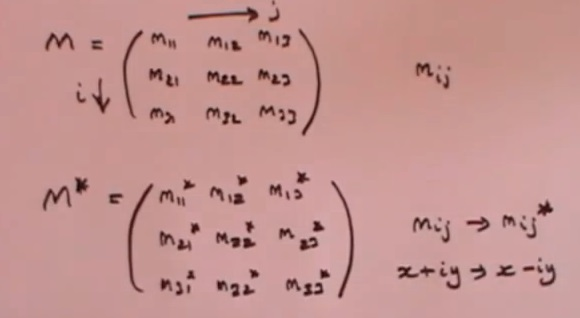18:40 When a matrix is the same as its Daggar - Hermitian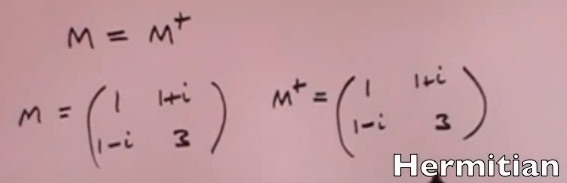20:00 Matrix applied to a Ket vector is new Ket vector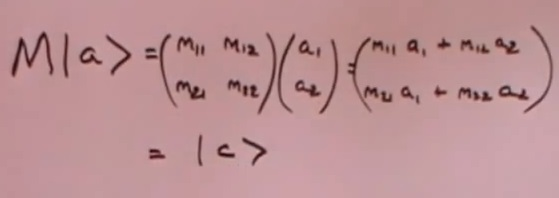24:57 Hermitian matrix, eigenvectors and eigenvalues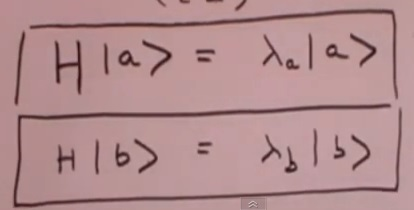27:04 Polarization - vertically polarized light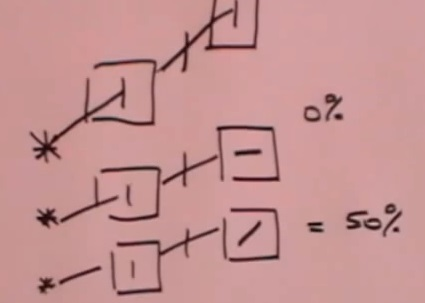33:20 Vertically polarized light through a horizontal polarizer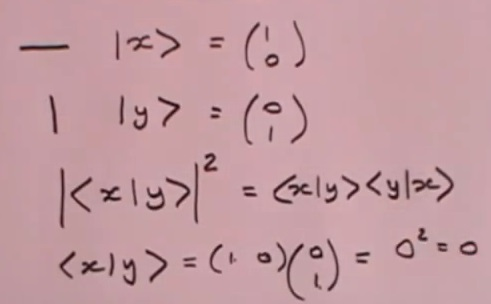40:10 Physical meaning of Hermitian, eigenvalues and eigenvectors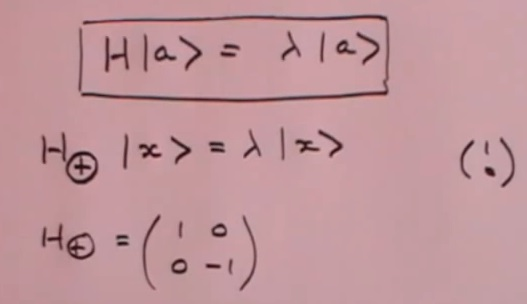44:10 Orthoganol vectors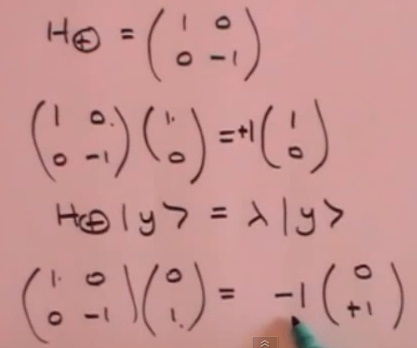46:30 Eigenvalues of experiment to test if light is horizontal or vertical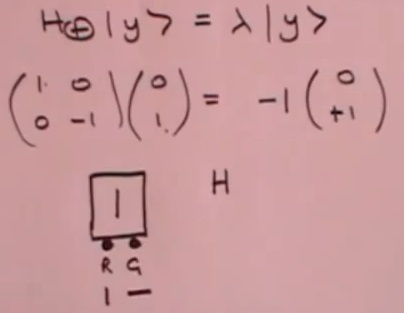48:40 Observable or experiment and state of system, will produce same state depending on polarization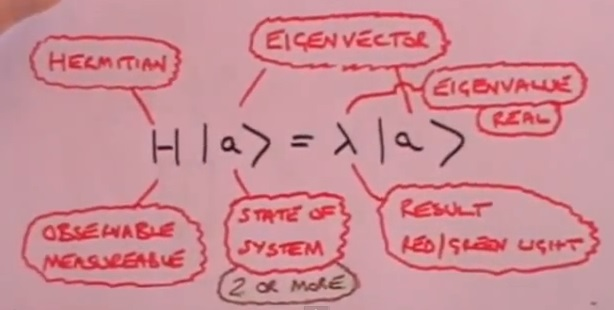50:00 45 degree light through a 45 degree polarizer and normalization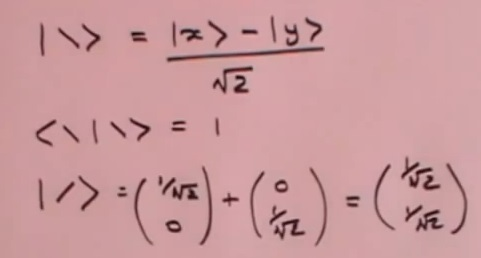61:00 45 degree light through a vertical polarizer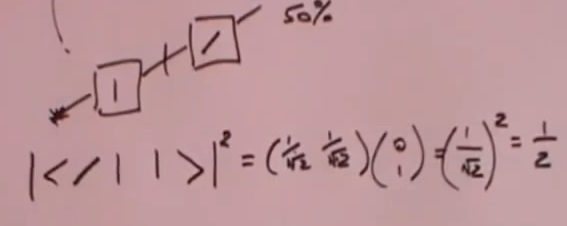64:00 Red light or green light on the detector